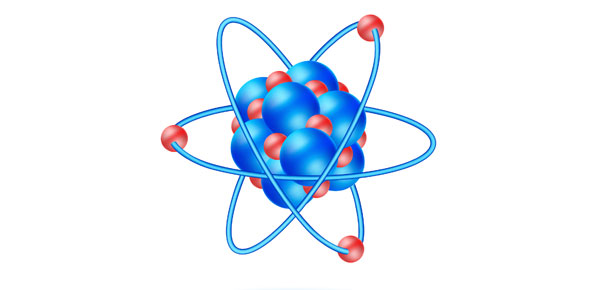# Holt Science Spectrum - Physical Science

42 Questions | Total Attempts: 46SettingsTo test knowledge of Chapter 2: Matter- Holt Science Spectrum- Physical Science

• 1.
What are the three states of Matter and give a brief definiton of each?
• 2.
Define three MAIN points of kinetic theory of matter.
• 3.
Give an example of sublimation
• 4.
Define chemical properties and give a list of some.
• 5.
Define physical properties and give a list of some.
• 6.
Define chemical change and give some examples.
• 7.
Define physical change and give some examples.
• 8.
What has fixed compositions and definite properties?
• A.

Mixture

• B.

Atoms

• C.

Pure substance

• D.

Molecules

• E.

Compounds

• 9.
What are represented with a one or two letter symbol?
• A.

Elements

• B.

Atoms

• C.

Molecules

• D.

Compounds

• E.

Mixtures

• 10.
Atoms do not make up elements.
• A.

True

• B.

False

• 11.
Which definition best describes what compounds are?
• A.

The smallest unit of a substance that exhibits all the properties of that substance

• B.

A combination of two or more elements bonded together

• C.

Two or more substances that can not mix into each other (ie. oil and water)

• D.

Substances that cannot be broken down into simpler substances

• E.

A combination of more than one pure substance

• 12.
____________ are the smallest unit of a substance that exhibits all the properties of that substance. (ie. Water)
• 13.
______________are matter and make up elements.
• 14.
The following are different types of mixtures...(you can select multiple answers)
• A.

Homogeneous

• B.

Miscible

• C.

Comogeneous

• D.

Heterogeneous

• E.

Immiscible

• 15.
Burning is a chemical property
• A.

False

• B.

True

• 16.
The three states of matter are solids, gases and liquids.
• A.

True

• B.

False

• 17.
What is the definition of evaporation?
• A.

The change of a substance from a gas to a liquid

• B.

The change of a substance from a liquid to a gas then to a liquid

• C.

The change of a substance from a solid to a gas then back to a solid

• D.

The change of a substance from a liquid to a gas

• 18.
What is the definition of condensation?
• A.

The change of a substance from a liquid to a gas then to a liquid

• B.

The change of a substance from a gas to a liquid

• C.

The change of a substance from a liquid to a gas

• D.

The change of a substance from a gas to a liquid

• 19.
What is the definition of sublimation?
• A.

The change of a substance from a solid to a gas

• B.

The change of a substance from a gas to a liquid

• C.

The change of a substance from a liquid to a gas

• D.

The change of a substance from a gas to a liquid

• 20.
What law states.....mass cannot be created or distroyed?
• A.

The Law of Conservation of Mass

• B.

The Law of Conservation of Energy

• 21.
What law states......energy cannot be created or destroyed?
• A.

The Law of Conservation of Mass

• B.

The Law of Conservation of Energy

• 22.
__________is the equation for density
• 23.
Changing state does changes composition and mass
• A.

True

• B.

False

• 24.
Read the definitions. Select the answer which list the words that corresond to the definitions.a) anything that has mass and occupies spaceb) the study of matter and how it changesc) the smallest unit of a substance that exhibits all of the property characteristic of that substanced) a substance made of atoms of more than one element bound togethere) a substance that cannot be broken down into simpler substancesf) Any matter that has a fixed composition and definite propertiesg)  a combination of more than one pure substanceh) describes 2 or more liquids that are able to dissolve into each other in various proportions (ie. water and methanol mix)i) describes 2 or more liquids that do NOT mix into each other (ie. water and oil do NOT mix into each other (ie.water and oil do NOT mix)j) the chemical symbols and numbers indicating the atoms contained in the basic unit of a substancek) the smallest particle that has the properties of an element
• A.

A)compound b)chemistry c)molecule d)matter e)element f) pure substance g) mixture h) miscible i) immiscible j) chemical formula k) atom

• B.

A) matter b)chemistry c)mixture d)compound e)element f) pure substance g) molecule h) miscible i) immiscible j) chemical formula k) atom

• C.

A) matter b)chemistry c)molecule d)compound e)immiscible f) pure substance g) mixture h) miscible i) element j) chemical formula k) atom

• D.

A) matter b)chemistry c)molecule d)compound e)element f) pure substance g) mixture h) miscible i) immiscible j) chemical formula k) atom

• 25.
Read the definitions. Select the answer which list the words that correspond to the definitions.a) the resistance of a fluid to flowb) the change of a substance from a gas to a liquidc) the change of a substance from a liquid to a gasd) the force exerted per unit area of a surfacee) the change of a substance from a solid to a gasf) the ability to change or move matter
• A.

A) viscosity b)evaporation c) sublimation d) energy e)condensation f)pressure

• B.

A) viscosity b)condensation c)evaporation d) pressure e)sublimation f) energy f)pressure

• C.

A) viscosity b)evaporation c)energy d) sublimation e)condensation f)pressure

• D.

A) viscosity b)evaporation c) sublimation d) energy e)pressure f)condensation

Related TopicsBack to top